# Concatenate If – Excel & Google Sheets

This tutorial will demonstrate how to concatenate cell values based on criteria using the CONCAT Function in Excel and Google Sheets.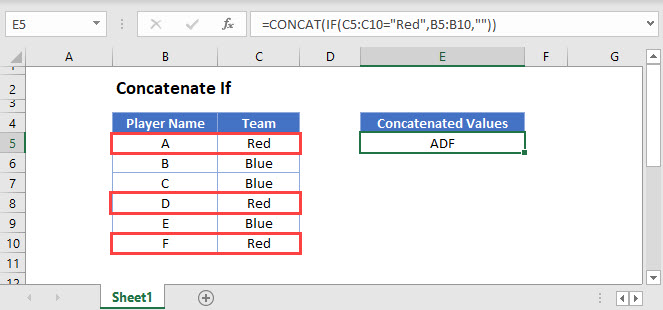## The CONCAT Function

Users of Excel 2019+ have access to the CONCAT Function which is used to join multiple strings into a single string.

Notes:

• Our first example uses the CONCAT Function and so is not available to Excel users before Excel 2019. See a later section in this tutorial for how to replicate this example in older versions of Excel.
• Google Sheets users also have access to the CONCAT Function, but unlike in Excel, it only allows two values or cell references to be joined together and does not allow inputs of cell ranges. See a later section on how this example can be achieved in Google Sheets by using the TEXTJOIN Function instead.

This example will use the CONCAT and IF Functions in an array formula to create a text string of Player Names which relate to a Team value of Red

``=CONCAT(IF(C3:C8="Red",B3:B8,""``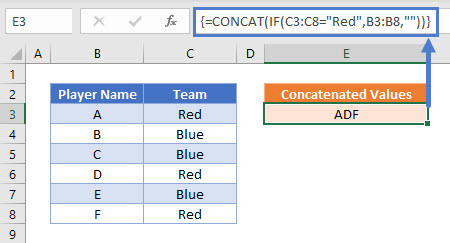Users of Excel 2019 will need to enter this formula as an array function by pressing CTRL + SHIFT + ENTER. Users of later versions of Excel do not need to follow this step.

To explain what this formula is doing, lets break it down into steps:

This is our final formula:

``=CONCAT(IF(C3:C8="Red",B3:B8,""``

First, Excel reads the range values into the formula:

``=CONCAT(IF({"Red"; "Blue"; "Blue"; "Red"; "Blue"; "Red"}="Red",{"A"; "B"; "C"; "D"; "E"; "F"},""``

Next the list of Team names is compared to the value Red:

``=CONCAT(IF({TRUE; FALSE; FALSE; TRUE; FALSE; TRUE},{"A"; "B"; "C"; "D"; "E"; "F"},""``

The IF Function replaces TRUE values with the Player Name, and FALSE values with “”

``=CONCAT({"A"; ""; ""; "D"; ""; "F"``

The CONCAT Function then combines all of the array values into one text string:

``="ADF"``

## Adding Delimiters or Ignoring Empty Values

If it is required to add delimiting values or text between each value, or for the function to ignore empty cell values, The TEXTJOIN Function can be used instead if you need to add delimiters

## Concatenate If – in pre-Excel 2019

As the CONCAT and TEXTJOIN Functions are not available before the Excel 2019 version, we need to solve this problem in a different way. The CONCATENATE Function is available but does not take ranges of cells as inputs or allow array operations and so we are required to use a helper column with an IF Function instead.

This next example shows how to use a helper column to create a text string of Player Names which relate to a Team value of Red:

``=IF(C3="Red",B3,"" &D4``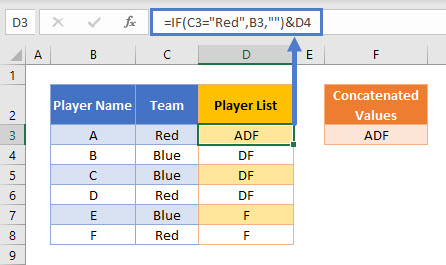The first step in this example is to use an IF Function to replicate the condition of Team = Red:

``=IF(C3="Red",B3,""``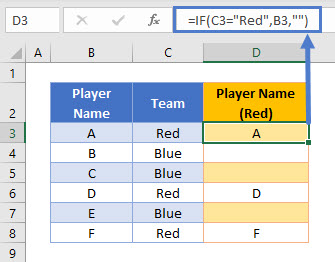Next, we can create a column that builds up a list of these values into one cell by also referencing the cell below it:

``=D3&E4``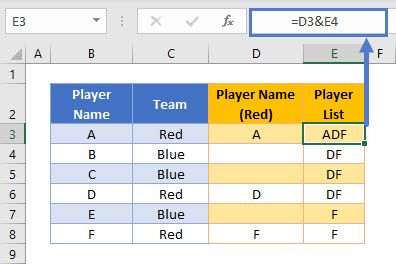This formula uses the & character to join two values together. Note that the CONCATENATE Function could be used to create exactly the same result, but the & method is often preferred as it is shorter and makes it clearer what action the formula is performing.

These two helper columns can then be combined into one formula:

``=IF(C3="Red",B3,""&D4``A summary cell can then reference the first value in the Player List helper column:

``=D3``

## Concatenate If in Google Sheets

Google Sheets users should use the TEXTJOIN Function to concatenate values based on a condition.

This example will use the TEXTJOIN and IF Functions to create a text string of Player Names which relate to a Team value of Red

``=ARRAYFORMULA(TEXTJOIN("",FALSE,IF(C3:C8="Red",B3:B8,"")))``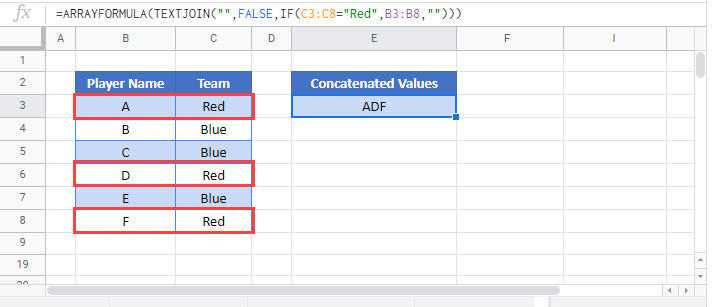As this formula requires array inputs for the cell ranges, the ARRAYFORMULA Function should be added to the formula by pressing CTRL + SHIFT + ENTER.

### Excel Practice Worksheet

Practice Excel functions and formulas with our 100% free practice worksheets!